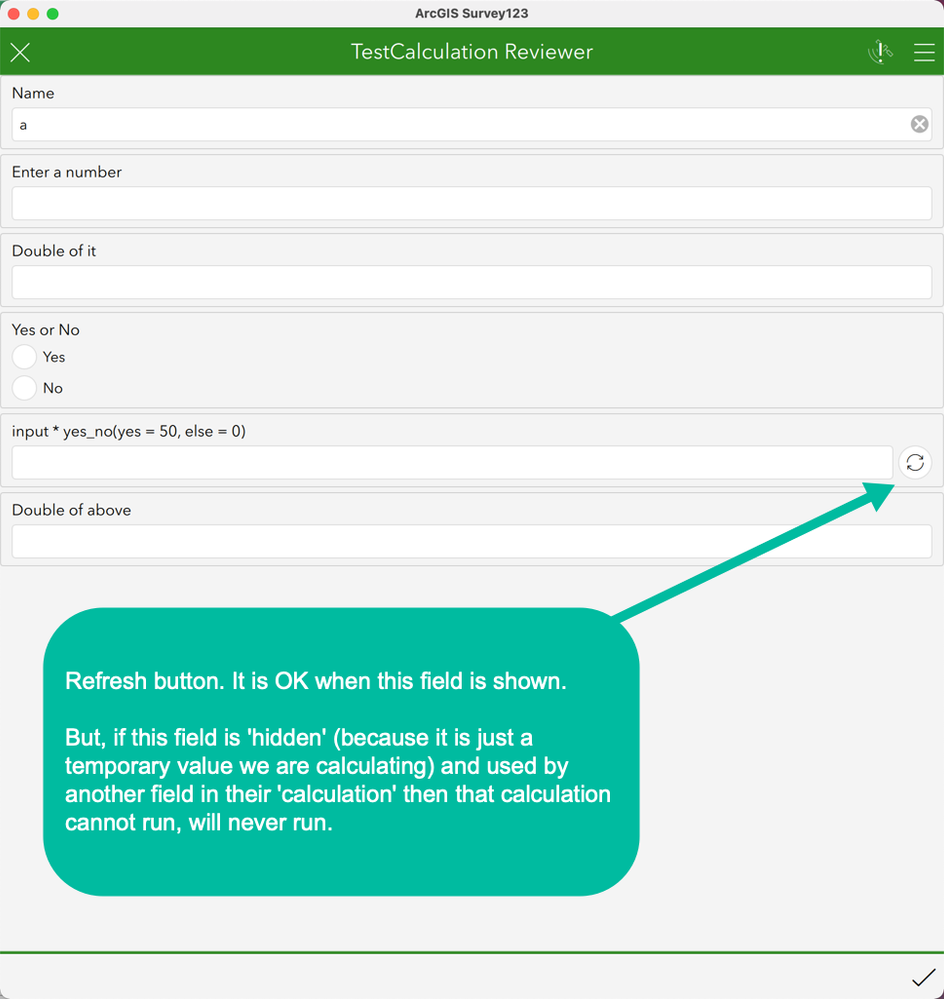# Do not require user to click refresh button when field is hidden

1019
5
05-14-2021 12:06 PMNew Contributor III

When using the Inbox feature and the 'calculation' column to calculate values, sometimes those calculated values are not now shown. Instead the user sees a recycling icon. When the user presses it then the value gets calculated.

This works OK, until that field is 'hidden' and its value is used by yet another 'calculation'. In these cases the final value cannot be calculated because there is no recycle button to press.1 Solution

Accepted Solutionsby
Occasional Contributor III

The thing that jumps out to me is the use of text fields for calculations. Have you considered using a combination of [type] note and calculate? See below:

 type name label calculation text name Name decimal input Enter a number calculate double_input \${input}*2 note Double of it: \${double_input} calculate yes_no_calc if(selected(\${yes_no},'yes'), \${input} * 50, 0) select_one yes_no yes_no Yes or No note grade \${yes_no_calc} calculate double_grade \${yes_no_calc}*2 note Double of above = \${double_grade}
5 Repliesby
Occasional Contributor III

I am likely not understanding what you are doing. Looks like you are using text fields, so I am not sure where your output is supposed to be. And sounds like you are using hidden fields for calculates?

Why not use a [type] calculate field? They are hidden by default. May help if you share your example doc.New Contributor III

I am using the calculation column to do some calculations. The above image is from the spreadsheet:

 type name label calculation text name Name decimal input Enter a number decimal double_input Double of it \${input}*2 select_one yes_no yes_no Yes or No decimal grade input * yes_no(yes = 50, else = 0) if(selected(\${yes_no}, 'yes'), \${input} * 50, 0) decimal double_grade Double of above \${grade}*2

The process is complicated:

To reproduce the problem you have to connect both TCSubmitter and TCReviewer to the TC Service. Turn on the Inbox for the TCReviewer. Then submit a TCSubmitter form with the number field empty. Then go to the Inbox of TCReviewer to see the submitted form.

The TCReviewer will show the "reload" icon above on that one field (see image above).

But, if I make that field hidden then the final field (double of the above) does not get the reload icon and does not get a value either.by
Occasional Contributor III

The thing that jumps out to me is the use of text fields for calculations. Have you considered using a combination of [type] note and calculate? See below:

 type name label calculation text name Name decimal input Enter a number calculate double_input \${input}*2 note Double of it: \${double_input} calculate yes_no_calc if(selected(\${yes_no},'yes'), \${input} * 50, 0) select_one yes_no yes_no Yes or No note grade \${yes_no_calc} calculate double_grade \${yes_no_calc}*2 note Double of above = \${double_grade}New Contributor III

You might say "just don't make the 'grade' field a 'hidden' field and the user will press the reload button".

The thing is, this is just a reproduction of the real spreadsheet, which has hundreds of values and dozens of intermediary values. 'grade' is an example of an intermediary value. We would like it to be hidden, but its value automatically calculated so that other calculations which depend on the value of 'grade' will also get calculated.New Contributor III

Yes, thanks!

Making the intermediary values  of type 'calculate' and the final values of type 'note' makes it work.